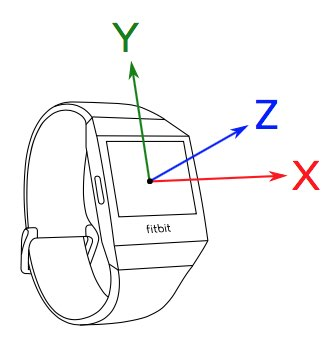Accelerometer Sensor Guide# Accelerometer Sensor Guide

## Overview

Accelerometers can be used to measure device acceleration, and determine orientation. The sensor measures acceleration along 3 orthogonal axes: X, Y and Z.

## A Brief Introduction to Axes

The X axis is parallel with the device's screen, aligned with the top and bottom edges, in the left-right direction. The Y axis is parallel with the device's screen, aligned with the left and right edges, in the top-bottom direction. The Z axis is perpendicular to the device's screen, pointing up.Acceleration includes the acceleration of gravity. So if the device is at rest, lying flat on a table, the acceleration along the Z axis should equal the acceleration of gravity (~9.8 m/s^2), and the acceleration along the X and Y axes should be 0.

The accelerometer axes readings are provided as floating point values, in units of m/s^2.

## Peeking the Current Accelerometer Reading

If you need to check the latest reading from the accelerometer, you can access the properties directly from the accelerometer object using the `onreading` event.

By default, the accelerometer sample rate is 100Hz (100 times per second).

``````import { Accelerometer } from "accelerometer";

if (Accelerometer) {
// sampling at 1Hz (once per second)
const accel = new Accelerometer({ frequency: 1 });
console.log(
`ts: \${accel.timestamp}, \
x: \${accel.x}, \
y: \${accel.y}, \
z: \${accel.z}`
);
});
accel.start();
}
``````

The Accelerometer API can also generate batches of data at a specified sample rate. This allows a developer to control the frequency that their application receives and processes sensor data.

The `onreading` event is emitted when a batch of readings is available, and the `.readings` property contains the sensor readings, with each data channel (`x`, `y`, `z`, and `timestamp`) as its own array.

By reducing the sample rate (`frequency`), or by increasing the batch size, developers will benefit from reduced CPU usage, and minimize their application's impact on battery life.

In order to use batched readings, the `batch` property must be specified during the initialization of the sensor.

``````import { Accelerometer } from "accelerometer";

if (Accelerometer) {
// the callback will be called once every two seconds
const accel = new Accelerometer({ frequency: 30, batch: 60 });
for (let index = 0; index < accel.readings.timestamp.length; index++) {
console.log(
);
}
}
accel.start();
}
``````

### Automatically Stopping and Starting

One of the best ways to conserve battery life is to stop the sensor when the display is off. You can use the Display API to respond to changes in the screen's power state.

``````import { Accelerometer } from "accelerometer";
import { display } from "display";

if (Accelerometer) {
const accel = new Accelerometer({ frequency: 1 });
console.log(
`ts: \${accel.timestamp}, \
x: \${accel.x}, \
y: \${accel.y}, \
z: \${accel.z}`
);
});
// Automatically stop the sensor when the screen is off to conserve battery
display.on ? accel.start() : accel.stop();
});
accel.start();
}
``````

## Accelerometer Best Practices

Here's a simple list of best practices to follow when using the Accelerometer API:

1. Always use the most optimal frequency for your specific needs.
2. Don't forget to call `accel.stop();` when you've finished using it.
3. Check if the sensor exists before using it.

## The Accelerometer in Action

If you're interested in using the Accelerometer API within your application, please review the Accelerometer API reference documentation.